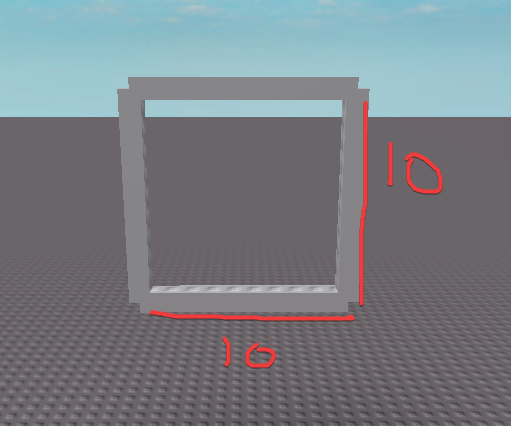# Using Math to generate Cuboid

1. What do you want to achieve?
I want to be able to generate Cuboid using math.

2. What is the issue?
I do not know the math used to generate Cuboid. I only know the math for a circle.

3. What solutions have you tried so far?
I have tried asking in various discords.

Circle Generation.

``````local Radius = 1
local Circle = math.pi * 2
local Amt = 10000

for Number = 1, Amt do
local Angle = Circle / Amt * Number
local X = math.sin(Angle) * Radius
local Z = math.cos(Angle) * Radius
end
``````

Sphere Generation

``````local function fibonacci_spiral_sphere(num_points)
local vectors = {}
local gr = (math.sqrt(5) + 1) / 2
local ga = (2 - gr) * (2 * math.pi)

for i = 1, num_points do
local lat = math.asin(-1 + 2 * i / (num_points + 1))
local lon = ga * i

local x = math.cos(lon) * math.cos(lat)
local y = math.sin(lon) * math.cos(lat)
local z = math.sin(lat)

table.insert(vectors, Vector3.new(x, y, z))
end

return vectors
end
``````

If anyone could help that would be nice (I’m trying to create a game like no man’s sky).

1 Like

This might sound real obvious, but why don’t you just make a smaller square, centre it to the middle of the main square, then hollow it out?

1 Like

Because I want the size layers etc to be customizable. Like this: Using Math to generate Spheres

Bumping because I still don’t know mans.

If the size of a square is a `number` called `Size` center of a square is a `Vector3` called `Center` then each corner of the square is defined as:

``````local Corner1 = Center + Vector3.new(Size / 2, Size / 2, 0)
local Corner2 = Center + Vector3.new(Size / 2, -Size / 2, 0)
local Corner3 = Center + Vector3.new(-Size / 2, -Size / 2, 0)
local Corner4 = Center + Vector3.new(-Size / 2, Size / 2, 0)
``````

Then you you can draw lines from each corner.

Final code could be something like this:

``````local function DrawLine(Position1, Position2)
local Distance = (Position1 - Position2).Magnitude

local Line = Instance.new("Part")

Line.Anchored = true
Line.Size = Vector3.new(1, 1, Distance)
--// This CFrame.new(P1, P2) overload is deprecated; but for simplicity I'll use it.
Line.CFrame = CFrame.new(Position1, Position2) * CFrame.new(0, 0, -Distance / 2)

return Line
end

local function DrawSquare(Center, Size)
local Square = Instance.new("Model")

local Corner1 = Center + Vector3.new(Size / 2, Size / 2, 0)
local Corner2 = Center + Vector3.new(Size / 2, -Size / 2, 0)
local Corner3 = Center + Vector3.new(-Size / 2, -Size / 2, 0)
local Corner4 = Center + Vector3.new(-Size / 2, Size / 2, 0)

local Line1 = DrawLine(Corner1, Corner2)
local Line2 = DrawLine(Corner2, Corner3)
local Line3 = DrawLine(Corner3, Corner4)
local Line4 = DrawLine(Corner4, Corner1)

Line1.Parent = Square
Line2.Parent = Square
Line3.Parent = Square
Line4.Parent = Square

Square.Parent = workspace

return Square
end

DrawSquare(Vector3.new(0, 15, 0), 10)
``````Thanks but that is not really what I meant I meant that I wanted to make a Cuboid not a square

Like I meant I 3D square not a 2D square